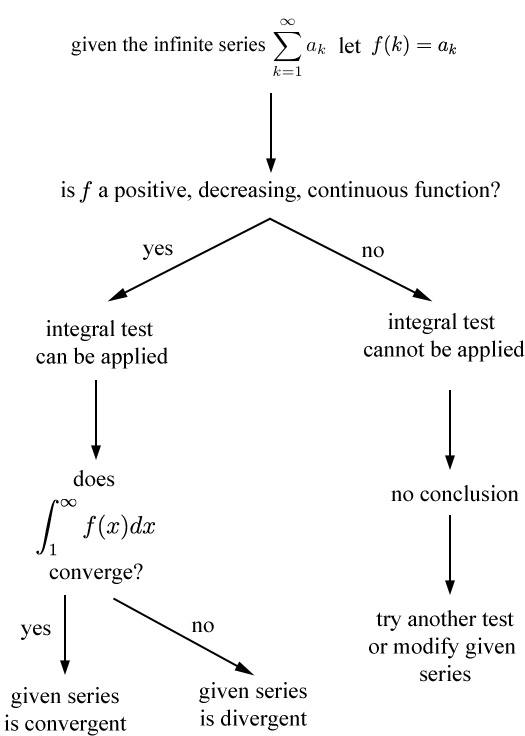# An Integral Test Flowchart

Previous: Theorem: The Integral Test

Next: Integral Test Example

The steps involved in applying the integral test to an infinite series are given in the flowchart below.This diagram shows the steps involved in conducting the integral test. Observe that in the case that when the integral test cannot be applied, we can try to perform some algebraic manipulation to apply the test. If for example, all terms in the series are negative, we can "pull out the negative sign" to make them positive.

Previous: Theorem: The Integral Test

Next: Integral Test Example